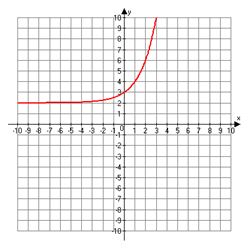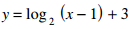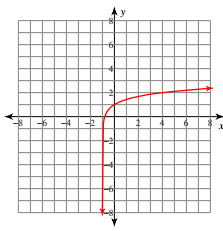#### MAT-HS.F-IF.07.e

 MAT-HS Targeted Standards(F) Concept: Function(IF) Domain: Interpreting FunctionsCluster: Analyze functions using different representations MAT-HS.F-IF.07* Graph functions expressed symbolically and show key features of the graph, by hand in simple cases and using technology for more complicated cases. a. Graph linear and quadratic functions and show intercepts, maxima, and minima. b. Graph square root, cube root, and piecewise-defined functions, including step functions and absolute value functions. c. Graph polynomial functions, identifying zeros when suitable factorizations are available, and showing end behavior. d. (+) Graph rational functions, identifying zeros and asymptotes when suitable factorizations are available, and showing end behavior. e. Graph exponential and logarithmic functions, showing intercepts and end behavior, and trigonometric functions, showing period, midline, and amplitude. f.  Graph f(x)=sin x and f(x)=cos x as representations of periodic phenomena. g.  (+) Graph trigonometric functions, showing period, midline, phase shift and amplitude

## Student Learning Targets:

• I can
• I can

• I can
• I can

### Skills (Performance) Targets

• Given an equation, I can graph a function and label all critical points, e.g. vertex, max or min value, x-intercepts, y-intercepts, slope.
• I can graph functions expressed symbolically and show key features of the graph (graph simple cases by hand and use technology to show more complicated cases).
• I can graph exponential functions, showing intercepts and end behavior.

• I can
• I can

## Proficiency Scale

 Score Description Sample Activity 4.0 In addition to Score 3.0, the student demonstrates in-depth inferences and applications regarding more complex material that go beyond end of instruction expectations. -Student is able to determine and describe intervals where the function is increasing and decreasing using interval notation. 3.5 In addition to Score 3.0 performance, the student demonstrates in-depth inferences and applications regarding the more complex content with partial success. 3.0 The student can: interprets and sketches key features of the linear graph (HS.F-IF.7a1) including: intercepts positive/negative slope interprets and sketches key features of the exponential graph (HS.F-IF.7b2) including: end behavior (growth/decay) interprets and sketches key features of the quadratic graph (HS.F-IF.7e) including: minimum/maximum axis of symmetry intercepts construct simple graphs by hand and use technology for more complicated cases. The student exhibits no major errors or omissions. Graph the function and identify the vertex, intercepts, and axis of symmetry.  y=x2-8x-7 Graph the function and identify the end behavior of the graph. y=(1/2)x 2.5 The student demonstrates no major errors or omissions regarding the simpler details and processes (Score 2.0 content) and partial knowledge of the more complex ideas and processes (Score 3.0 content). 2.0 There are no major errors or omissions regarding the simpler details and processes as the student can: recognize and recall basic terminology, such as: linear equation. quadratic equation. exponential equation. slope intercepts minimum maximum end behavior growth and decay symmetry axis of symmetry only interprets and sketches some of the key features of the graph. can identify if a function is linear, exponential, or quadratic However, the student exhibits major errors or omissions regarding the more complex ideas and processes. Graph the set of points and determine the relationship.  (i.e. linear, exponential, or quadratic)   (0, 0), (1, 1), (-1, -0.5), (2, 3) 1.5 The student demonstrates partial knowledge of the simpler details and processes (Score 2.0 content) but exhibits major errors or omissions regarding the more complex ideas and procedures (Score 3.0 content). 1.0 With help, the student demonstrates a partial understanding of some of the simpler details and processes (Score 2.0 content) and some of the more complex ideas and processes (Score 3.0 content). - 0.5 With help, the student demonstrates a partial understanding of some of the simpler details and processes (Score 2.0 content) but not the more complex ideas and processes (Score 3.0 content). 0.0 Even with help, the student demonstrates no understanding or skill. -

## Alg II Graph Exponential Functions Proficiency Scale

 Score Description Sample Activity 4.0 (advanced) In addition to Score 3.0, the student demonstrates in-depth inferences and applications regarding more complex material that go beyond end of instruction expectations. Write an exponential function whose graph lies between the graphs of y=(0.5)x and y=(0.25)x+3 3.5 In addition to Score 3.0 performance, the student demonstrates in-depth inferences and applications regarding the more complex content with partial success. 3.0 (proficient) The student can: graph functions expressed symbolically and show key features of the graph (graph simple cases by hand and use technology to show more complicated cases). graph exponential functions, showing intercepts and end behavior (asymptote). The student exhibits no major errors or omissions. Graph the following equations and identify all the key features including: x-intercepts, y-intercepts, increasing interval, decreasing interval, domain, range and asymptote.2.5 The student demonstrates no major errors or omissions regarding the simpler details and processes (Score 2.0 content) and partial knowledge of the more complex ideas and processes (Score 3.0 content). 2.0 (progressing) There are no major errors or omissions regarding the simpler details and processes as the student can: recognize and recall basic terminology, such as: x-intercepts y-intercepts intervals (decreasing and increasing) asymptote domain range However, the student exhibits major errors or omissions regarding the more complex ideas and processes. Given the graph, identify all the key features including: x-intercepts, y-intercepts, increasing interval, decreasing interval, domain and range and asymptote.1.5 The student demonstrates partial knowledge of the simpler details and processes (Score 2.0 content) but exhibits major errors or omissions regarding the more complex ideas and procedures (Score 3.0 content). 1.0 (beginning) With help, the student demonstrates a partial understanding of some of the simpler details and processes (Score 2.0 content) and some of the more complex ideas and processes (Score 3.0 content). 0.5 With help, the student demonstrates a partial understanding of some of the simpler details and processes (Score 2.0 content) but not the more complex ideas and processes (Score 3.0 content). 0.0 Even with help, the student demonstrates no understanding or skill.

## Alg II Graph Logarithmic Functions Proficiency Scale

Score   Description Sample Activity

4.0

In addition to Score 3.0, the student demonstrates in-depth inferences and applications regarding more complex material that go beyond end of instruction expectations. Once a hurricane reaches land, the wind speed s (in knots) within the hurricane is related to the time t (in hours) the hurricane remains over land.  For one particular hurricane, this relationship can be modeled  by the equation y= -57.1 log t +121. Graph the equation and analyze the graph to determine how long the hurricane must be over land for winds speeds to become about 80 knots.
3.5 In addition to Score 3.0 performance, the student demonstrates in-depth inferences and applications regarding the more complex content with partial success.

3.0

(proficient)

The student can:
• Graph logarithmic functions expressed symbolically and show key features of the graph (graph simple cases by hand and use technology to show more complicated cases).

The student exhibits no major errors or omissions.

Graph the following equation and identify all the key features including: increasing interval, decreasing interval, asymptote, domain and range.2.5 The student demonstrates no major errors or omissions regarding the simpler details and processes (Score 2.0 content) and partial knowledge of the more complex ideas and processes (Score 3.0 content).

2.0

(progressing)

There are no major errors or omissions regarding the simpler details and processes as the student can:
• recognize and recall basic terminology, such as:
• logarithm
• base
• exponent
• intervals (increasing and decreasing)
• asymptote
• domain
• range

However, the student exhibits major errors or omissions regarding the more complex ideas and processes.

 Given the graph, identify all the key features including the asymptote, increasing and decreasing intervals, domain, and range.1.5 The student demonstrates partial knowledge of the simpler details and processes (Score 2.0 content) but exhibits major errors or omissions regarding the more complex ideas and procedures (Score 3.0 content).

1.0

(beginning)

With help, the student demonstrates a partial understanding of some of the simpler details and processes (Score 2.0 content) and some of the more complex ideas and processes (Score 3.0 content).
0.5 With help, the student demonstrates a partial understanding of some of the simpler details and processes (Score 2.0 content) but not the more complex ideas and processes (Score 3.0 content).
0.0 Even with help, the student demonstrates no understanding or skill.

## Resources

 Web Vocab intercepts maximum asymptote minimum exponential function